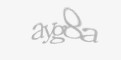0
0
0
s2sdefault

### Article Index

I have been having connectivity issues of a strange variety at home. I have called the ISP and had many discussions, and frankly, some argumants on my connectivity to the internet. The ISP, like all others, claims that it isn't their fault, and must be something with my wireless router, or other problem with my network and my setup. Myself, knowing exactly how this is all setup, I know this to be not the case. Or at least I am very reasonably sure...

Since there is debate over the source of the failure, I have written a little script that does a couple of pings, and logs the results to a file. In the end, there are 2 pings, one sent to the default gateway, and one sent to google.ca.This will help narrow down any possible failures... If I am able to ping google.ca, the internet connectivity is fine. If I can ping my default gateway, but not google, then the internet connection itself is the problem. And if I cannot ping the gateway, then there's the problem there. Again, none of this is very useful without any date/time data, so we need that. And since I am using a ping, I also want to get the return time to serve as a (minor) basis for connectivity speed.

There are probably many other ways to accomplish the same goals I am after here, but since I have the ability to do so, I wrote up my own script for this. I have tested this script here in my lab for a few days, and I think it works as expected. Though only a little more "real-world" testing will help dis/prove this. Let's take a look at the script:

`#! /bin/bash`

`PING_TARGET="google.ca"`

`LOG_DIR=/var/log/conn`

`LOG_FILE=\$LOG_DIR"/conn.log"`

`PING_COMMAND="ping -W 1 -c 1"`

`if [ "`id -u`" != "0" ]`

`then`

`    echo "You need root privileges!"`

`    exit`

`fi`

`if [ ! -e \$LOG_DIR ]`

`then`

`    mkdir \$LOG_DIR`

`fi`

`if [ ! -e \$LOG_FILE ]`

`then`

`    touch \$LOG_FILE`

`    chmod 757 \$LOG_FILE`

`    echo "Date/Time, Uptime (in secs), CPU Load, Next Hop IP, Ping Time (in ms), Target IP, Ping Time (in ms)," >> \$LOG_FILE`

`fi`

`COUNTER=1`

`while [ \$COUNTER -le 6 ]`

`do`

`    FINAL_LOG=\$(date),`

`    UPTIME=\$(cat /proc/uptime | awk '{ print \$1 }') # uptime in secs`

`    FINAL_LOG+=\$UPTIME,`

`    CPU=\$(cat /proc/loadavg | awk '{print \$1}')`

`    FINAL_LOG+=\$CPU,`

`    DEFAULT_ROUTE=\$(/sbin/route | grep default | awk '{ print \$2 }')`

`    wait`

`    if [ -z "\$DEFAULT_ROUTE" ]`

`    then`

`        FINAL_LOG+="No Default Route, ,";`

`    else`

`        PING_DR="\$(\$PING_COMMAND \$DEFAULT_ROUTE 2>&1)"`

`        DR_IP=\$(awk -F " " '{print \$3;}' <<< \$PING_DR) `

`        FINAL_LOG+=\$DR_IP,`

`        DRPINGTIME=\$(awk -F " " '{print \$14;}' <<< \$PING_DR)`

`        DRPINGTIME=\${DRPINGTIME:5}`

`        FINAL_LOG+=\$DRPINGTIME,`

`    fi`

`    PING_OUTPUT="\$(\$PING_COMMAND \$PING_TARGET 2>&1)"`

`    if [[ \$PING_OUTPUT == *"unknown host"* ]]`

`    then`

`        FINAL_LOG+="DNS ERROR, ,";`

`    elif [[ \$PING_OUTPUT == *"0 received"* ]]`

`    then`

`        FINAL_LOG+="Ping Timeout, ,";`

`    else`

`        PING_IP=\$(awk -F " " '{print \$3;}' <<< \$PING_OUTPUT) `

`        FINAL_LOG+=\$PING_IP,`

`        PINGTIME=\$(awk -F " " '{print \$15;}' <<< \$PING_OUTPUT)`

`        PINGTIME=\${PINGTIME:5}`

`        FINAL_LOG+=\$PINGTIME,`

`    fi`

`    echo \$FINAL_LOG >> \$LOG_FILE`

`    COUNTER=\$(( COUNTER+1 ))`

`    sleep 10`

`done`

Let's break the script down...